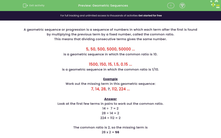# Find the Missing Terms in Geometric Sequences

In this worksheet, students will find the missing term in geometric sequences.Key stage:  KS 3

Curriculum topic:   Algebra

Curriculum subtopic:   Use Geometric Sequences

Popular topics:   Algebra worksheets

Difficulty level:#### Worksheet Overview

A geometric sequence or progression is a sequence of numbers in which each term after the first is found by multiplying the previous term by a fixed number, called the common ratio.

This means that dividing consecutive terms gives the same number.

5, 50, 500, 5000, 50000 ...

is a geometric sequence in which the common ratio is 10.

1500, 150, 15, 1.5, 0.15 ...

is a geometric sequence in which the common ratio is 1/10.

Example

Work out the missing term in this geometric sequence:

7, 14, 28, ?, 112, 224 ...

Look at the first few terms in pairs to work out the common ratio.

14 ÷  7 = 2

28 ÷ 14 = 2

224 ÷ 112 = 2

The common ratio is 2, so the missing term is

28 x 2 = 56

Let's practise this in the following questions.

### What is EdPlace?

We're your National Curriculum aligned online education content provider helping each child succeed in English, maths and science from year 1 to GCSE. With an EdPlace account you’ll be able to track and measure progress, helping each child achieve their best. We build confidence and attainment by personalising each child’s learning at a level that suits them.

Get started